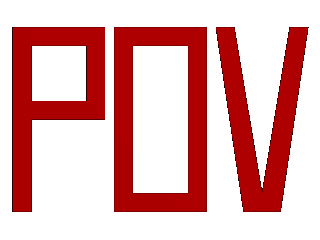POV-Ray : Documentation : 1.3.2.3 Polygon ObjectPOV-Ray 3.6 Documentation Online View#### 1.3.2.3 Polygon Object

The `polygon` object can be used to create any planar, n-sided shapes like squares, rectangles, pentagons, hexagons, octagons, etc.

A polygon is defined by a number of points that describe its shape. Since polygons have to be closed the first point has to be repeated at the end of the point sequence.

In the following example we will create the word "POV" using just one polygon statement.

We start with thinking about the points we need to describe the desired shape. We want the letters to lie in the x-y-plane with the letter O being at the center. The letters extend from y=0 to y=1. Thus we get the following points for each letter (the z coordinate is automatically set to zero).

Letter P (outer polygon):

```    <-0.8, 0.0>, <-0.8, 1.0>,
<-0.3, 1.0>, <-0.3, 0.5>,
<-0.7, 0.5>, <-0.7, 0.0>
```

Letter P (inner polygon):

```    <-0.7, 0.6>, <-0.7, 0.9>,
<-0.4, 0.9>, <-0.4, 0.6>
```

Letter O (outer polygon):

```    <-0.25, 0.0>, <-0.25, 1.0>,
< 0.25, 1.0>, < 0.25, 0.0>
```

Letter O (inner polygon):

```    <-0.15, 0.1>, <-0.15, 0.9>,
< 0.15, 0.9>, < 0.15, 0.1>
```

Letter V:

```    <0.45, 0.0>, <0.30, 1.0>,
<0.40, 1.0>, <0.55, 0.1>,
<0.70, 1.0>, <0.80, 1.0>,
<0.65, 0.0>
```

Both letters P and O have a hole while the letter V consists of only one polygon. We will start with the letter V because it is easier to define than the other two letters.

We create a new file called ` polygdem.pov` and add the following text.

```  camera {
orthographic
location <0, 0, -10>
right 1.3 * 4/3 * x
up 1.3 * y
look_at <0, 0.5, 0>
}
light_source { <25, 25, -100> color rgb 1 }
polygon {
8,
<0.45, 0.0>, <0.30, 1.0>, // Letter "V"
<0.40, 1.0>, <0.55, 0.1>,
<0.70, 1.0>, <0.80, 1.0>,
<0.65, 0.0>,
<0.45, 0.0>
pigment { color rgb <1, 0, 0> }
}
```

As noted above the polygon has to be closed by appending the first point to the point sequence. A closed polygon is always defined by a sequence of points that ends when a point is the same as the first point.

After we have created the letter V we will continue with the letter P. Since it has a hole we have to find a way of cutting this hole into the basic shape. This is quite easy. We just define the outer shape of the letter P, which is a closed polygon, and add the sequence of points that describes the hole, which is also a closed polygon. That is all we have to do. There will be a hole where both polygons overlap.

In general we will get holes whenever an even number of sub-polygons inside a single polygon statement overlap. A sub-polygon is defined by a closed sequence of points.

The letter P consists of two sub-polygons, one for the outer shape and one for the hole. Since the hole polygon overlaps the outer shape polygon we will get a hole.

After we have understood how multiple sub-polygons in a single polygon statement work, it is quite easy to add the missing O letter.

Finally, we get the complete word POV.

```  polygon {
30,
<-0.8, 0.0>, <-0.8, 1.0>,    // Letter "P"
<-0.3, 1.0>, <-0.3, 0.5>,    // outer shape
<-0.7, 0.5>, <-0.7, 0.0>,
<-0.8, 0.0>,
<-0.7, 0.6>, <-0.7, 0.9>,    // hole
<-0.4, 0.9>, <-0.4, 0.6>,
<-0.7, 0.6>
<-0.25, 0.0>, <-0.25, 1.0>,  // Letter "O"
< 0.25, 1.0>, < 0.25, 0.0>,  // outer shape
<-0.25, 0.0>,
<-0.15, 0.1>, <-0.15, 0.9>,  // hole
< 0.15, 0.9>, < 0.15, 0.1>,
<-0.15, 0.1>,
<0.45, 0.0>, <0.30, 1.0>,    // Letter "V"
<0.40, 1.0>, <0.55, 0.1>,
<0.70, 1.0>, <0.80, 1.0>,
<0.65, 0.0>,
<0.45, 0.0>
pigment { color rgb <1, 0, 0> }
}
```